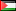##### Numerical Treatment of Strongly Elliptic Integral Equation
Publication Type
Original research
Authors
• Naji Qatanani
Fulltext

The numerical treatment of boundary integral equations in the form of boundary element methods has became very popular and powerful tool for engineering computations of boundary value problems, in addition to finite difference and finite element methods. Here, we present some of the most important analytical and numerical aspects of the boundary integral equation. The concept of the principle symbol allows the characterization of the boundary integral equation whose variational formulation on the boundary provides there a Gãrding inequality. Therefore, the Galerkin method can be analyzed similarly to the domain finite element methods providing asymptotic convergence if the number of grid points increases. These asymptotic error analysis will be presented in details. To illustrate the efficiency of the Galerkin boundary element method we consider as an numerical experiment the strongly elliptic boundary integral equation with the logarithmic single layer potential. Consequently, we use the Gaussian elimination method as a direct solver and the conjugate gradient iteration to solve the positive definite linear system.  A comparison is drawn between these methods.

Journal
Title
Journal of Vectorial Relativity JVR 5 (2010) 2 26-38
Publisher
--
Publisher Country
PalestinePublication Type
Both (Printed and Online)
Volume
--
Year
2010
Pages
--